# Methods To Check The Performance Of Classification Models

Classification problems are one of the most used categories of problem statements in Machine Learning and Data Science. When we explore the real-life application where Machine Learning and Data Science is being used by the tech giants like Google, Apple, Tesla, Microsoft, Facebook, etc., we will find that 9 out of 10 problem statements are classification problem statements. Because of its popularity, many new methods are coming every day and challenge the previously existing methods.

BUT ON WHAT BASIS?

The answer is simple, on the accuracy grounds. Research papers also publish the work and compare their newer approaches’ results with the already benchmarked research papers. So whenever we say that we have built a machine learning model, the first question that comes to us is, “What is the accuracy of your model?

To test our classification model, there are many methods based on which we can say that our machine learning model is better. In addition, there are some of the task specialized metrics to evaluate the model as well. But in this article, we will talk about the most popular methods used to check performance and are widely used in the machine learning and data science industries.

1. Accuracy and its limitations
2. Confusion Matrix
3. Precision & Recall
4. F1-Score
5. Specificity
6. Receiver Operating Characteristic Curve (ROC)
7. Area Under Curve (AUC)

Let’s start understanding every method in detail.

### Accuracy

Accuracy for a classification problem is a straightforward calculation and is widely used in industries. In mathematical representation,

Accuracy = ((Number of correct predictions)/(Number of total predictions))100*

As we know, in classification problems, our machine learning model categorizes the input variables into different classes. We calculate the total number of classifications our model made and how many classifications are correct

``````from sklearn.metrics import accuracy_score
#Y_pred is the predicted target variable and
#Y_act is the true target variable. Then,
print("Accuracy = ", accuracy_score(Y_act, Y_pred))
``````

#### Limitations of Accuracy

Although it is a very widely used metric, it has some serious limitations. Suppose you have trained a model to classify the images into two classes, “Cat” or “No-Cat”. You tested your model on 100 images containing cats, and your model gave the value corresponding to the “Cat” class all the time. So accuracy from the above formulae, ((100)/(100)*100) = 100%. Wow!!!

But what if, your model is always predicting the “Cat” class only?

Here is the catch. If you had tested your model over 100 images having no cats, then accuracy would be ((0)/(100)*100) = 0%, but you already have stated everywhere that your model has achieved 100% accuracy. So we must not judge the model by just accuracy metric as it works well with a balanced dataset but not with the unbalanced one.

### Confusion Matrix

One of the best evaluation metrics that can be considered as the base for other performance measurements. It is called a confusion matrix as it confuses the users very often.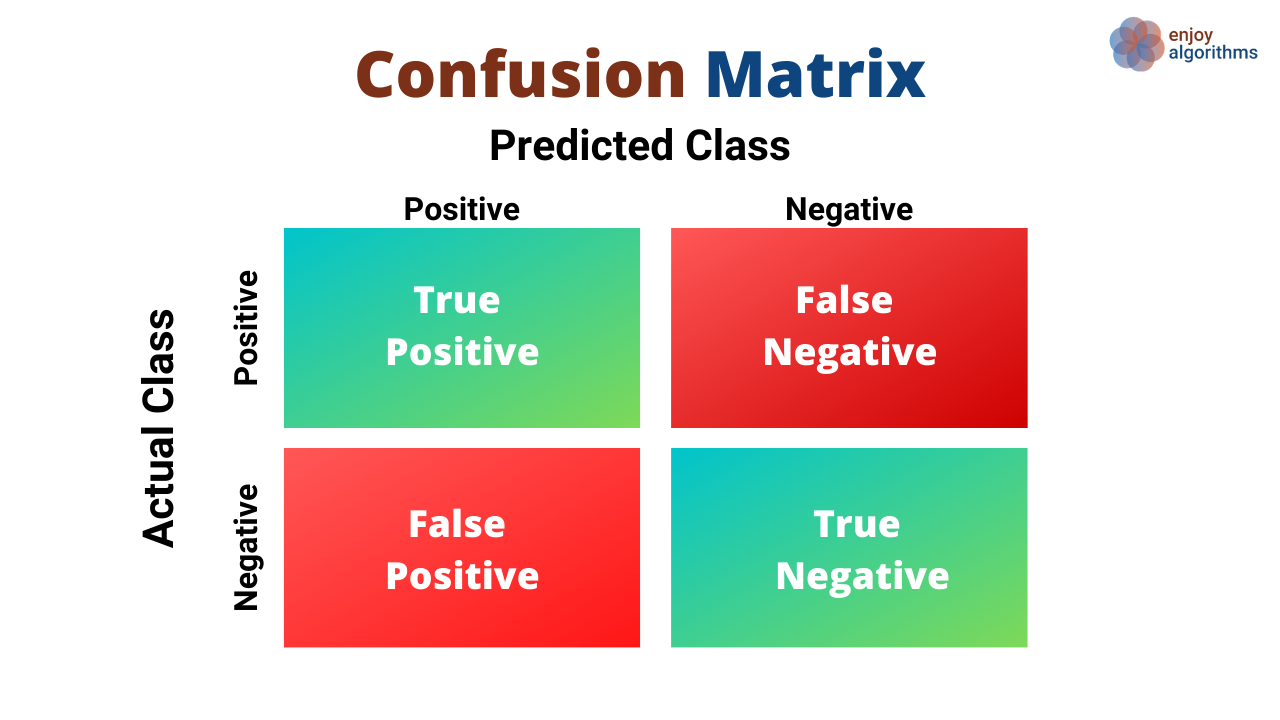Let’s take an example to learn the four terms, True Positive (TP), True Negative (TN), False Positive (FP), and False Negative (FN), which constitute the confusion matrix. All the performance measures will be defined using these terms.

Suppose we want to eat an apple, and as we are health conscious, we built a machine learning classification model that takes the apple's image as input and predicts whether it is fresh or old. Luckily, we have our own farm, and we know which apple belongs to the fresh category and which apples don’t. Based on this, let’s define four important terms.

True Positive (TP): This is when our model says an apple to be fresh and that apple was actually fresh.

True Negative (TN): This is when our model says an apple to be old and that apple was actually old.

False Positive (FP): This is when our machine learning model says an apple to be fresh, but in reality, that apple was old. This case is popularly known as Type I Error.

False Negative (FN): This is when our machine learning model says an apple to be old but in reality, that apple was fresh. This case is popularly known as Type II Error.

``````from sklearn.metrics import confusion_matrix
y_true = ["fresh", "old", "old", "fresh", "fresh", "fresh"]
y_pred = ["fresh", "old", "fresh", "old", "fresh", "old"]
arr = confusion_matrix(y_true, y_pred, labels=["fresh", "old"])
print("Confusion Matrix = ",arr)
tn, fp, fn, tp = arr.ravel()
``````

Now, if we have to re-define the accuracy term, then,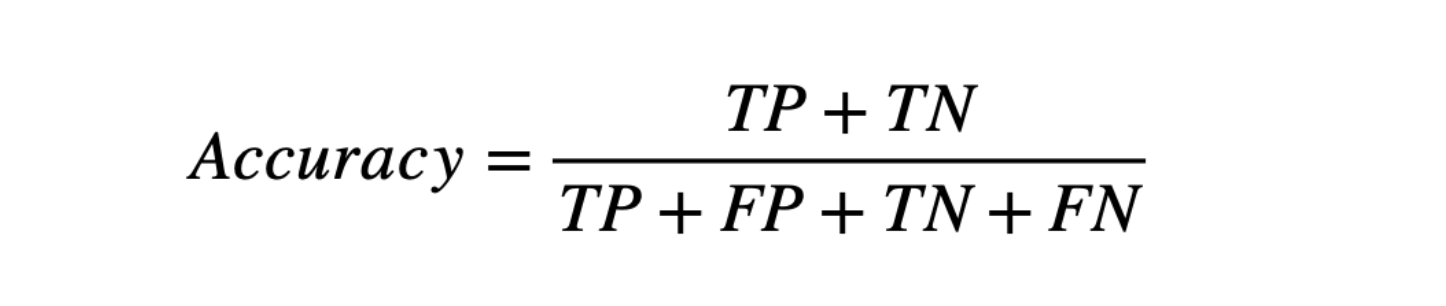which states, Out of all the apples we have, how many were correctly predicted as fresh and old.

### Precision & Recall

Now, suppose there is a scenario where we have 100 apples. We predicted these apple types using our two different classification models; they segregated the apples as “fresh” and “old”. When we observed the predictions, we found,

Model 1 : TP = 68, FN = 22, FP = 0, TN = 10.

Model 2 : TP = 90, FN = 0, FP = 4, TN = 6.

Now, suppose it's our farm, and we want to store the apple. But the problem is if we store old apples and fresh ones together, it will convert “fresh into “old” ones. In such a scenario, we must be penalizing False Positives because, in such a scenario, it will convert all the fresh ones into old ones. Precision is a measure for that only. In the above case, Model 1 is preferred.Now take another scenario, we want to sell the apple as soon as possible to make extra profit. In such a scenario, there is no problem of mixing up, but the goal is to penalize the False Negatives so that more and more apples getting predicted as fresh. A recall is a measure of that only. In the above case, Model 2 is preferred.Note: Recall is also called Sensitivity and True Positive Rate (TPR)

### F1-Score

Now, suppose we have just started a supermarket, where the customers are less initially. We want a balance between the storage of apples and the sales of apples because we don’t know how many days it will take to finish all the stock. In this scenario, we need to pick the model with a higher F1-Score.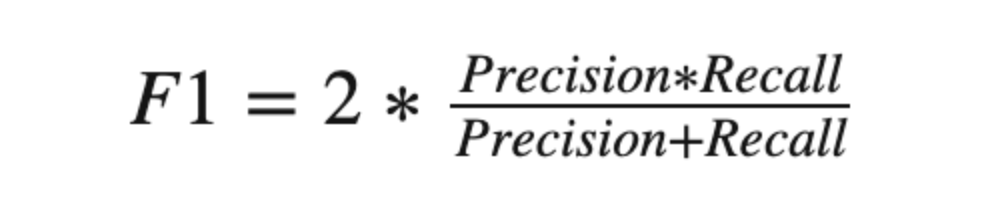### Specificity

Suppose we want our model to be perfectly sure about the old apples to eliminate them from our stock. In such a scenario, we will try to penalize the False Positives and make our model surely predict the “older” ones. Specificity gives us a measure of that.### ROC

If we are familiar with the binary classification methodology, we must know that our model predicts probability. In our example earlier, suppose the model receives the image of an apple and predicts that it is “fresh” with the probability of X%. If the value of X is greater than the threshold value, then we say that model predicted the apple as “fresh”; otherwise, we say that model predicted that apple as “old”. But what if we change that threshold?

We plot True Positive Rate (TPR)/Recall/Sensitivity as our Y-axis and False Positive Rate (FPR) as our X-axis for a varying threshold value. We can clearly say that Model 3 is better than Model 2, and Model 2 is better than Model 1 as we are increasing the True positive rate.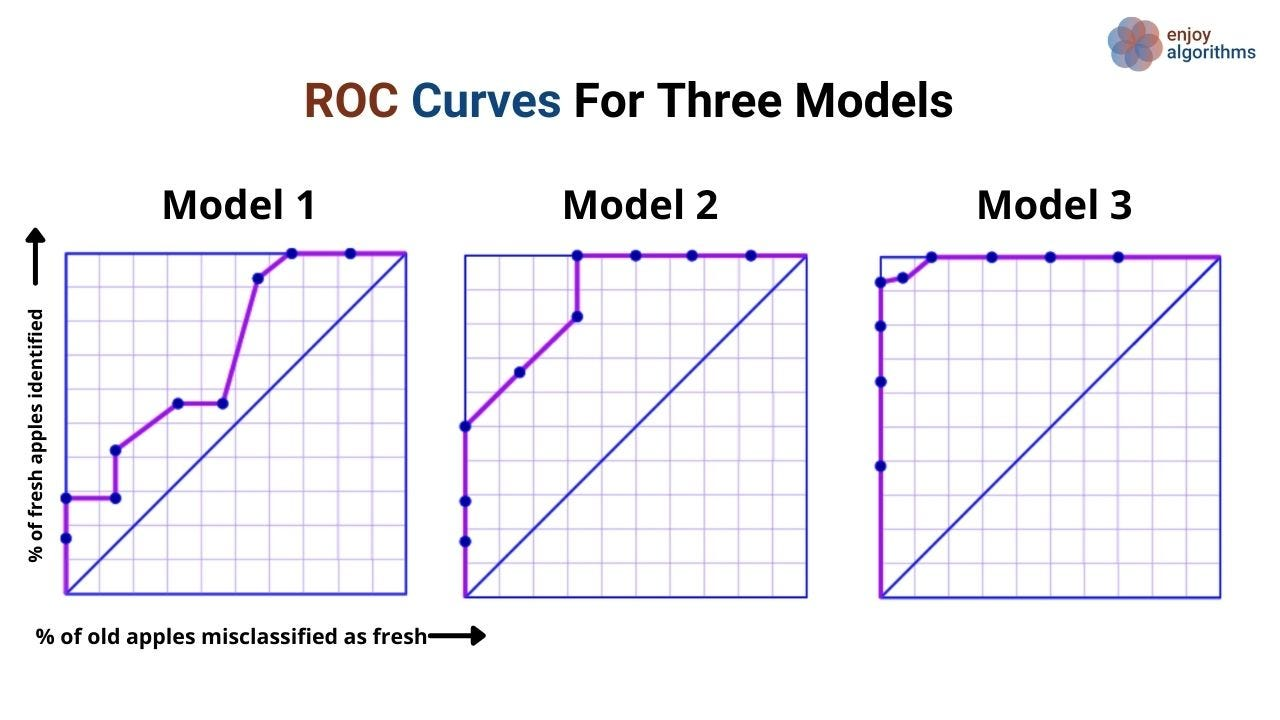### AUC

Suppose we built a random model to classify our apples as fresh and old. It has a 50% probability to perform the classification, and hence the line as x=y in the above image has an area under the curve as 0.5. If we have built a perfect model, then our model would classify our apples with 100% probability, and here, AUC = 1. So we can say that “More the area under the curve, the better the model”.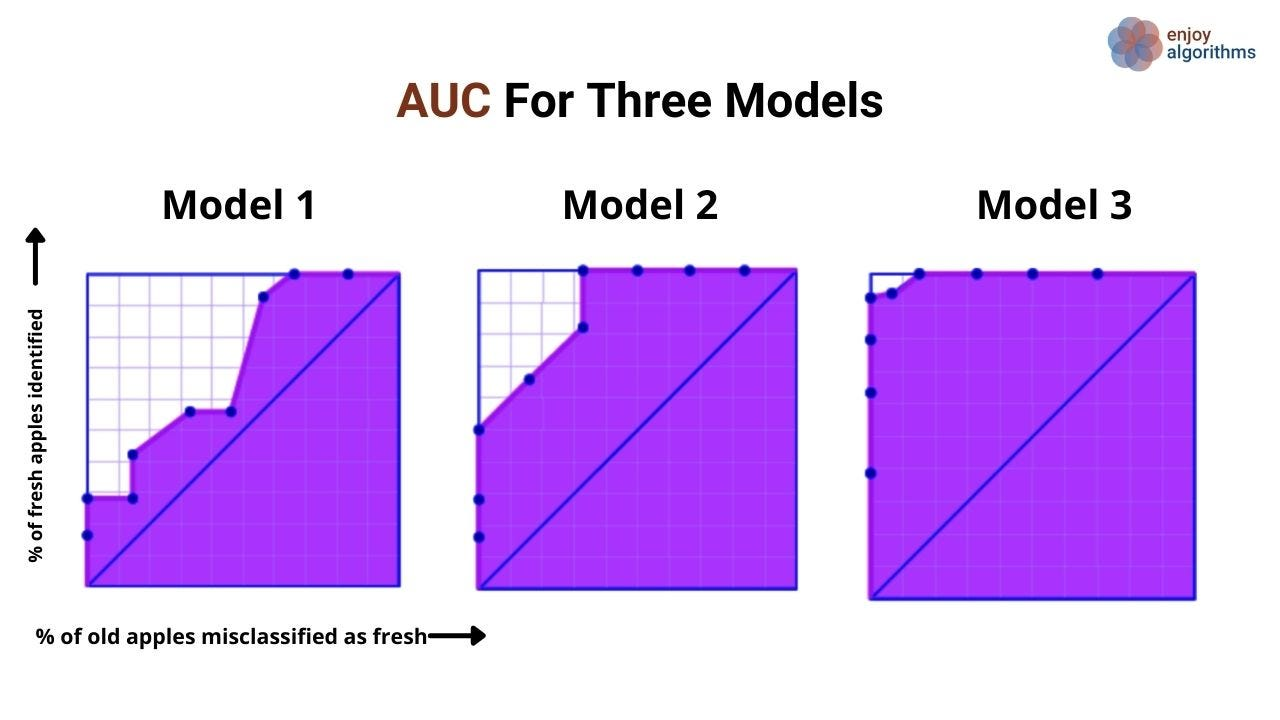For a better understanding, we can have a look at the below image.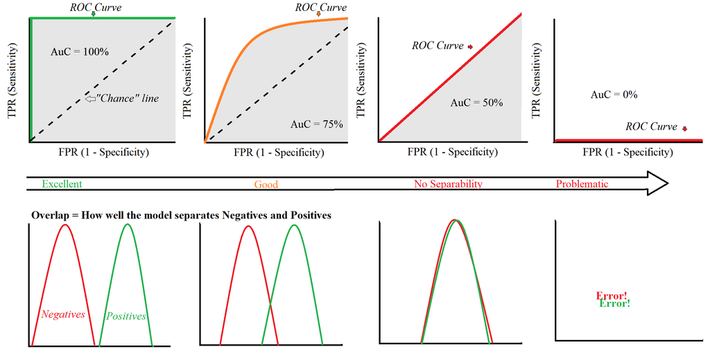Source: Data Science Central

### Possible Interview Questions

Generally, questions on the evaluation metric are asked when we have represented our models’ performance using any of the following metrics. But knowing the answers to the following questions will surely help.

1. What evaluation metrics should be used for your project?
2. What are the problems that accuracy terms can suffer?
3. What is the confusion matrix, and why is it considered as a base for all other metrics?
4. Is it always preferred to have a better F1 score rather than better precision or recall?
5. What is the ROC plot, name X and Y-axis? Note: This is frequently asked.

### Conclusion

This article has covered Accuracy, Confusion Matrix, Precision, Recall, F1-Score, ROC, and AUC, which are the most frequently used evaluation metric for the classification models. There can be other evaluation methods, but we have tried to cover the most frequent ones. We have covered every matric with a beautiful story of fresh and old apples. We hope you must have enjoyed it.

### Enjoy Learning! Enjoy Evaluating! Enjoy Algorithms!

Get well-designed application and interview centirc content on ds-algorithms, machine learning, system design and oops. Content will be delivered weekly.